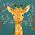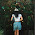## Angelica's Proportion Problems

1) If 5 pencils cost 20 cents, how much would 15 pencils cost?

Let x be the cost of pencils (15 of them)
Word ratio, ratio table, find x (cross product)

The cost of 15 pencils, would be 60 cents.

2) Darrell drove 187 miles in 3 hours. What was Darrell's average rate of speed in miles per hour?

Let x be the average rate of speed in one hour
Word ratio, ratio table, find x (cross product)

Darrell's average speed rate in an hour is  62.3 miles.

A word problem for you:

The ratio of 7th graders to 8th graders participating in the science fair is 4:3. There are 18 eighth graders participating in the science fair. How many seventh graders are there?
6:49 PM

#### 2 comments :

1.~There would be 24 7th graders. (I got the answer by doing cross multiplication.)

2.